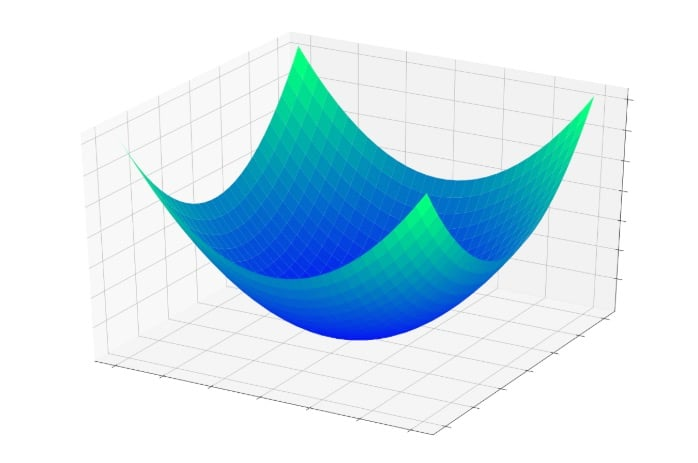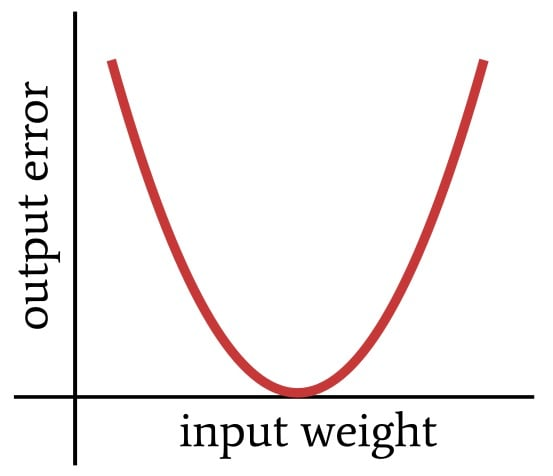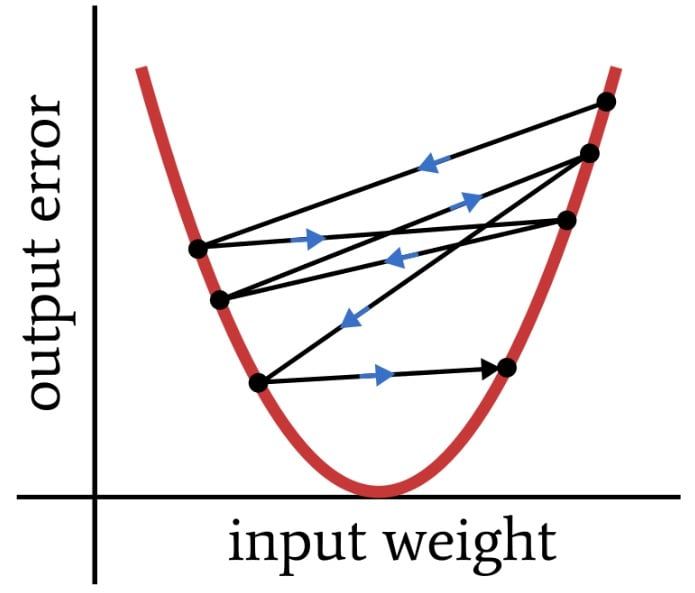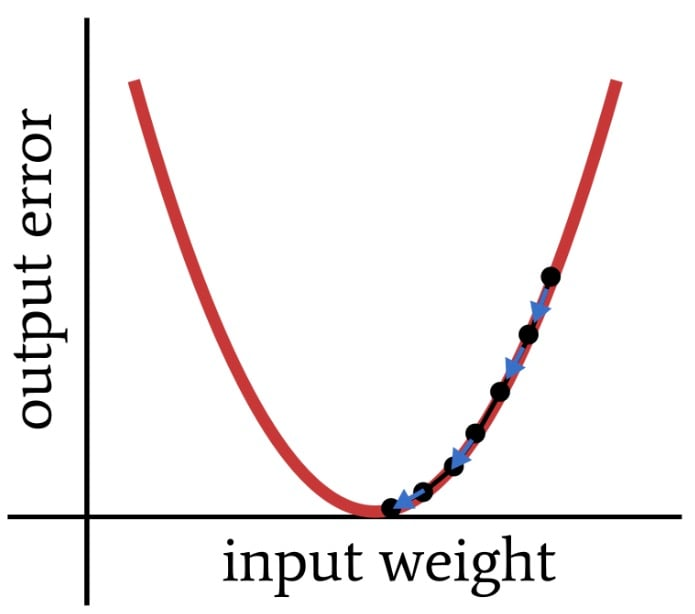Technical Article

# Understanding Learning Rate in Neural Networks

December 19, 2019 by Robert Keim

## This article discusses learning rate, which plays an important role in neural-network training.

Welcome to AAC's series on neural networks. Check out the series below to learn about Perceptron neural networks and training theory for neural networks overall:

In this article, we'll discuss the concept of learning rate and explore how it can affect neural network training.

### What Is Learning Rate?

As you may have guessed, learning rate influences the rate at which your neural network learns. But there’s more to the story than that.

First, let’s clarify what we mean by “learning.” In the context of neural networks, “learn” is more or less equivalent in meaning to “train,” but the perspective is different. An engineer trains a neural network by providing training data and performing a training procedure. While this is happening, the network is learning—or more specifically, it is learning to approximate the input–output relationship contained in the training data. The manifestation of learning is weight modification, and learning rate affects the way in which weights are modified.

### Minimizing Error in Neural Networks

The previous article introduced the concept of the error bowl—that is, a three-dimensional surface that helps us to visualize the process by which a node’s error gradually decreases toward zero as its input weights are modified during training.If we have a point whose location is determined by the values of the two weights and the node’s output error, each weight modification causes the point to jump to a different location somewhere on this error surface. These jumps tend toward the bottom of the bowl, where error is minimized; they don’t lead directly to minimum error because each training sample is only a small piece of the mathematical puzzle.

### The Effect of Learning Rate

Learning rate influences the size of the jumps that lead to the bottom of the bowl. I’m going to switch to a two-dimensional representation now because the images will be easier to create and easier to interpret. Here is our two-dimensional error function:As you might recall from a previous article, we used the following learning rule to update the weights:

$w_{new} = w+(\alpha\times\delta\times input)$

where $$\alpha$$ is the learning rate and $$\delta$$ is the difference between expected output and calculated output (i.e., the error). Every time we apply this learning rule, the weight jumps to a new point on the error curve. If $$\delta$$ is large, those jumps could also be quite large, and the network may not train effectively because the weights are not gradually converging toward minimum error. Instead, they’re bouncing around somewhat chaotically, as shown below.##### Large weight jumps are not conducive to good convergence.

Since $$\delta$$ is multiplied by learning rate before the modification is applied to the weight, we can reduce the size of the jumps by choosing $$\alpha$$ < 1. The goal is to use learning rate to promote moderately fast, consistent convergence.

The sort of training that we want might look something like this:### How to Choose the Learning Rate

There’s no universal rule that tells you how to choose a learning rate, and there’s not even a neat and tidy way to identify the optimal learning rate for a given application. Training is a complex and variable process, and when it comes to learning rate, you have to rely on intuition and experimentation.

If your network can process the training data quickly, you can simply choose a few different learning rates and compare the resulting weights (if you know what the weights should be) or input fresh data and assess the relationship between learning rate and classification accuracy.

A more involved approach, and one that would be more practical for networks that require long training times, is to analyze the changes in error as the network is training. The error should be decreasing toward the minimum, and the changes in error should be small enough to avoid the “bouncing” behavior shown above but not so small that the network learns extremely slowly. Like so many other things in life, learning rate is about balance.

There is much more that could be said about how to find an optimal learning rate, and maybe we’ll explore this issue more thoroughly in a future article. But not today.

### Learning Rate Schedules

Before we finish up, I want to briefly discuss a learning technique that is, I believe, an important tool for those who are working with neural networks. The first thing to recognize is that the learning rate does not need to be constant throughout the entire training procedure. Learning rate is applied every time the weights are updated via the learning rule; thus, if learning rate changes during training, the network’s evolutionary path toward its final form will immediately be altered.

One way to take advantage of this is to decrease the learning rate during training. This is called “annealing” the learning rate. There are various ways to do this, but for now, the important thing is to recognize why it helps.

When the network first starts training, the error is probably going to be large. A higher learning rate helps the network to take long strides toward minimum error. As the network approaches the bottom of the error curve, though, these long strides can impede convergence, similar to how a person taking long strides might find it difficult to land directly in the middle of a small circle painted on the floor. As learning rate decreases, long strides become smaller steps, and eventually the network is tiptoeing toward the center of the circle.

### Conclusion

I warned you in the first article that this would be a long series. This is installment number 6, and the end is not yet in sight. In any case, I hope that you enjoyed this explanation of learning rate. In the next article, we’ll discuss the issue of “local minima.”